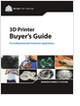Formula HomeFinanceFinancial TermsMoney FactorCalculatorsLoan (typical)Loan (advanced)Canadian MortgageLease (typical)Lease (advanced)APR (typical)APR (advanced)ResourcesBibliography
STEM Career Outlook

Wages, employment opportunities, and growth projections for STEM jobs.

Selecting the Right 3D Printer

Discover how to choose the right 3D printer for your needs and the key performance attributes to consider.

Learn the best principles to negotiate the salary you deserve!

CNC Machining Design Guide

more free publicationsAPR CalculatorLoan Amount (C):  *Extra Cost (E):  Interest Rate % (R):  No. of Months (N):  APR (A):  * The Extra Cost (E) is the lump sum of all extra costs involved in the loan, which include points, application fee, closing cost, processing fee, title fee, and so on. In short, it's the money you borrowed that you never saw. This calculator first calculates the monthly payment using C+E and the original interest rate r = R/1200:The APR (a = A/1200) is then calculated iteratively by solving the following equation using the Newton-Raphson method:APR ExplainedAnnual Percentage Rate (APR) is the equivalent interest rate considering all the added costs to a given loan. Naturally, it is a function of the loan amount, the interest rate, the total added cost, and the terms. The APR would equal the interest rate if there is no additional costs to a given loan. Here is an example:Total money borrowed:(The actual amount of money you are going to receive from the lender.) \$200,000 Total extra cost:(Points, application fee, closing cost, processing fee, title fee, and so on.) \$5,000 Interest rate: 7.5% Term: 30 yearsTo find the monthly payment for this loan, we can use the Loan Calculator Scenario #1 with \$205,000 as the total loan amount (you are not borrowing this much but you will owe this amount when the loan is closed), 7.5% as the interest rate, and 360 as the number of payments (1 payment/month for 30 years). The monthly payment is found to be \$1,433.39.

Now, since you are only borrowing \$200,000 but paying \$1,443.39/month for 30 years, we can back-calculate the equivalent interest rate (APR) by using the Loan Calculator Scenario #2. The answer is,

Annual Percentage Rate = 7.75%.

The APR Calculator on this page will perform these two calculations in one pass.

GlossarySTEM Career Outlook

Wages, employment opportunities, and growth projections for STEM jobs.Selecting the Right 3D Printer

Discover how to choose the right 3D printer for your needs and the key performance attributes to consider.